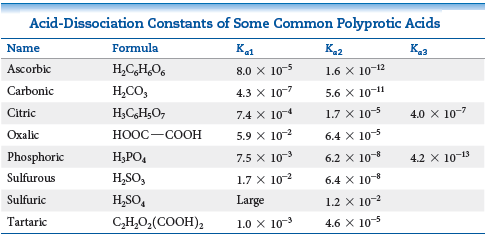# Problem: Using the table below, how many of the following salts are expected to produce acidic solutions: NaHSO4, NaHC2O4, NaH2PO4, and NaHCO3?

🤓 Based on our data, we think this question is relevant for Professor Wade's class at OSU.

###### FREE Expert Solution

Given: NaHSO4, NaHC2O4, NaH2PO4, and NaHCO3

The cation in all of the given salts is Na+: Na+ is a main group metal cation with a +1 charge  Na+ is a neutral ion

Therefore, we have to look at the anion.  Compare Ka and Kb:

Recall that

$\overline{){{\mathbf{K}}}_{{\mathbf{w}}}{\mathbf{=}}{{\mathbf{K}}}_{{\mathbf{a}}}{\mathbf{×}}{{\mathbf{K}}}_{{\mathbf{b}}}}$

The Kb of S2 is:

$\overline{){{\mathbf{K}}}_{{\mathbf{b}}}{\mathbf{=}}\frac{{\mathbf{K}}_{\mathbf{w}}}{{\mathbf{K}}_{\mathbf{a}\mathbf{2}}}}$

For NaHSO4: NaHSO4 dissociates to form Na+ and HSO4.

Anion: HSO4

Ka2 of H2SO4 = 1.2 × 10–2

The Kb of HSO4 is:

${\mathbf{K}}_{\mathbf{b}}\mathbf{=}\frac{\mathbf{1}\mathbf{.}\mathbf{0}\mathbf{×}{\mathbf{10}}^{\mathbf{-}\mathbf{14}}}{\mathbf{1}\mathbf{.}\mathbf{2}\mathbf{×}{\mathbf{10}}^{\mathbf{-}\mathbf{2}}}$

Kb = 8.33 × 10–2

Ka Kb

NaHSO4 will produce an acidic solution.

For NaHC2O4: NaHC2O4 dissociates to form Na+ and HC2O4.

Anion: HC2O4

Ka2 of H2C2O4 = 6.4 × 10–5###### Problem Details

Using the table below, how many of the following salts are expected to produce acidic solutions: NaHSO4, NaHC2O4, NaH2PO4, and NaHCO3?